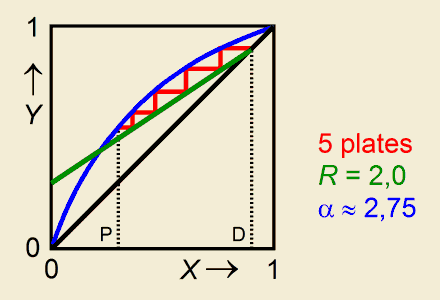# Batch Rectification Calculation of the Number of Platesa program for the Texas Instruments TI-83and TI-84 (Plus) graphing calculators

### Distillation at a given distillate composition

The boiling liquid is a mixture of two volatile components, wherein the mole fraction of the more volatile component is symbolized by X (hence 1–X matches the less volatile component).
Given values for:
• the relative volatility α,
• the composition XD of the drawn off distillate,
• the composition XP of the boiling mixture in the pot,
• and the reflux ratio R,
the program calculates the number of theoretical plates in the distillation column inclusive of the pot as well as all other liquid and vapour compositions X and Y belonging to the theoretical plates (Y is the mole fraction of the more volatile component in a vapour phase).
The calculations are according to the McCabe-Thiele method.### Program Listing

Elucidation and Help
PROGRAM:RECTIBP Title
ClrHome > PRGM > I/O > ClrHome
Disp "REL.VOLATILITY" (α>1)
Input A
max(A,1)→A > MATH > NUM > max(
"→" Symbolizes the STO> key
Disp "X DISTILLATE" Composition of the distillate. X is the mole fraction of the more volatile component of the liquid (0<X<1).
Input D
min(D,1)→D > MATH > NUM > min(
Disp "X POT MIXTURE" Composition of the boiling mixture. X is the mole fraction of the more volatile component (0<X<1).
Input M
min(M,D)→M
Disp "REFLUX RATIO"
Input R
0→Z:ClrHome
Lbl 1 > PRGM > CTL > Lbl
0→N:D→X
While X>M
(RX+D)/(R+1)→Y Y is the mole fraction of the more volatile component in the vapour phase (0<Y<1).
Y/(Y+A(1–Y))→X Y and X are at equilibrium
N+1→N
If Z=0
Goto 2
N→[B](N,1) > 2nd, MATRX > NAMES > [B]
X→[B](N,2)
Y→[B](N,3)
Lbl 2
End
If Z=1
Goto 3
Disp "NUMBER OF PLATES",N
{N,3}→dim([B]) > 2nd, MATRX > MATH > dim(
1→Z
Goto 1
Lbl 3
Disp "","PLATE, X, Y  >>"
Pause
round([B],2)→[B]
Disp [B] Compositions (first seven plates)
Pause :ClrHome
Disp "X,Y IN MAT [B]" Merely a reminder

After running and quitting the program, you still can have access to the liquid and vapour compositions X and Y of all plates. This may especially be of intrest when the number of plates exceeds seven. You'll find them stored in matrix [B] (> 2nd, MATRX > NAMES > [B], ENTER, ENTER).

### Example

Consider a mixture of benzene and toluene.

Input values:
• relative volatility = 2,55
XD (distillate) = 0,97
XP (boiling mixture in the pot) = 0,60
• reflux ratio = 4

Output:
Number of theoretical plates = 4 (that is 3 plates in the column)
Plate    X         Y
1       0,93     0,97       (top of the column)
2       0,85     0,94
3       0,73     0,87
4       0,58     0,78       (pot)

The vapour with composition Y3 = 0,87 is ascending from plate 3.
X3 = 0,73 is the composition of the liquid on plate 3. Both phases are at equilibrium.

(To retrieve the plate compositions as shown in an example coming with the program RECTIBD, put in XD = 0,972.)

Hein ten Horn
Top | Programs Texas Instruments | Home